Related Articles

# How to Set the X and the Y Limit in Matplotlib with Python?

• Last Updated : 02 Dec, 2020

Prerequisites : Matplotlib

In this article, we will learn how to set the X limit and Y limit in matplotlib with Python. Before that some concepts need to be familiarized with:

• Python : Python is a high-level, general-purpose and a very popular programming language. It is being used in web development, Machine Learning applications, along with all cutting edge technology in Software Industry.
• Matplotlib : Matplotlib is a visualization library in supported by Python for 2D plots of arrays. Matplotlib is a multi-platform data visualization library built on NumPy arrays and designed to work with the broader SciPy stack. It was introduced by John Hunter in the year 2002.
• xlim() : It is a function in pyplot module of matplotlib library which is used to get or set the x-limits of the current axes.
• ylim() : It is a function in pyplot module of matplotlib library which is used to get or set the y-limits of the current axes.

## Approach

• Import Library
• Plot a graph
• Use xlim() / ylim() or both.

Let’s discuss these steps with the help of some examples :

Example 1: (By using xlim() method)

## Python3

 `# import packages``import` `matplotlib.pyplot as plt``import` `numpy as np`` ` `# create data``x ``=` `np.linspace(``-``10``, ``10``, ``1000``)``y ``=` `np.sin(x)`` ` `# plot the graph``plt.plot(x, y)`` ` `# limit x by -5 to 5``plt.xlim(``-``5``, ``5``)`

Output :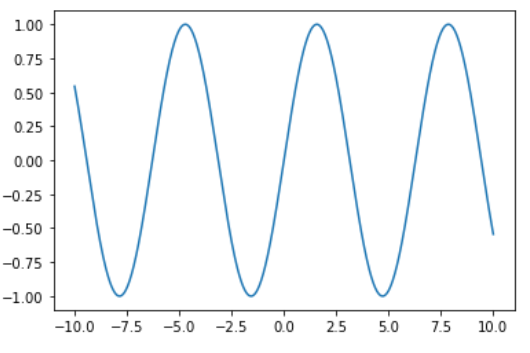Simple Plot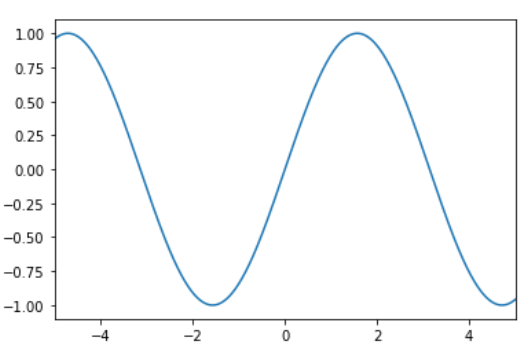X limited Plot

Example 2: (By using ylim() method)

## Python3

 `# import packages``import` `matplotlib.pyplot as plt``import` `numpy as np`` ` `# create data``x ``=` `np.linspace(``-``10``, ``10``, ``1000``)``y ``=` `np.cos(x)`` ` `# plot the graph``plt.plot(x, y)`` ` `# limit y by 0 to 1``plt.ylim(``0``, ``1``)`

Output :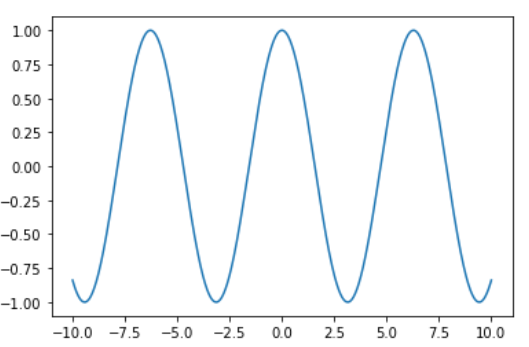Simple Plot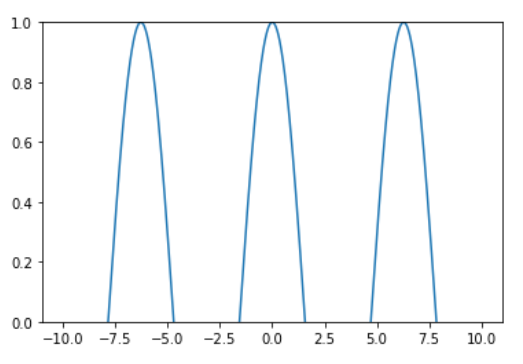Y Limited Plot

Example 3: (Using both xlim() and ylim() methods)

## Python3

 `# import packages``import` `matplotlib.pyplot as plt``import` `numpy as np`` ` `# create data``x ``=` `np.linspace(``-``10``, ``10``, ``20``)``y ``=` `x`` ` `# plot the graph``plt.plot(x, y)`` ` `# limited to show positive axes``plt.xlim(``0``)``plt.ylim(``0``)`

Output :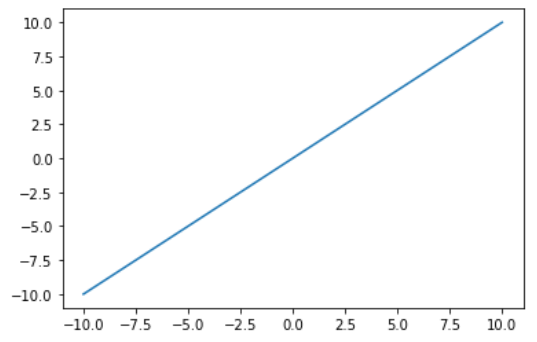Simple Plot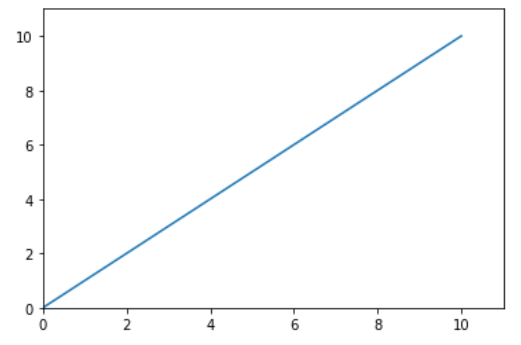X and Y Limited Plot

Attention geek! Strengthen your foundations with the Python Programming Foundation Course and learn the basics.

To begin with, your interview preparations Enhance your Data Structures concepts with the Python DS Course. And to begin with your Machine Learning Journey, join the Machine Learning – Basic Level Course

My Personal Notes arrow_drop_up